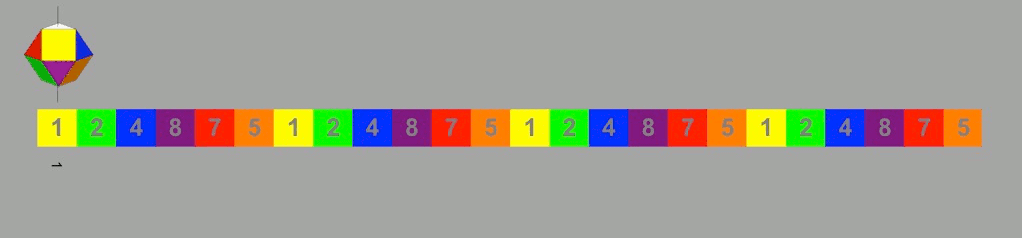The grand aim of all science is to cover the greatest number of empirical facts by logical deduction from the smallest possible number of hypotheses or axioms.
Albert Einstein
New Release!

Elements of Dimensional Geometry:
mechanics of spherical harmonic spatial/temporal proportion

In his new book, P.S. Williams refines and elaborates on the theorems postulated in his first book, Natural Number. It is structured as an axiomatic extension of Euclid’s elements relevant to the geometry of proportion.In his first book, Natural Number, P.S. Williams links the dimensional geometry of continuous proportion with modulus-9 complementary number symmetry and a novel prime number theory by combining Euclidean geometric principles with data-mined fragments of ancient Pythagorean number theory: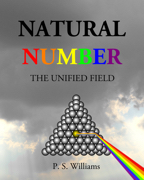I present a compelling series of geometric, mathematical, and symmetric proofs and observations that serve to objectively substantiate my contention that, at its most fundamental quantum levels, energy is best characterized as a quantized multidimensional waveform sustained and propagated by the cooperative spatial/temporal dynamics of the golden ratio and it’s negative reciprocal – the spatial base and temporal anti-base of continuous golden proportion.
In an astonishing revelation, Williams provides objective mathematical, geometric, and symmetric proofs of a highly predictive alternative to Schrodinger’s 3D spherical coordinate based harmonic model — a model based on a spatial/temporal interpretation of Pascal’s triangle as a dimensional continuum of quantized energy states!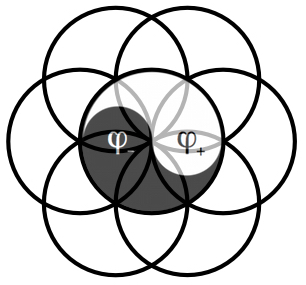Ultimately I find that when the geometry of Pascal’s triangle is interpreted in a spatial-temporal dimensional context and analyzed with complementary modulus-9 symmetry, super-symmetric spatial-temporal cuboctahedral dipole fields are revealed that provide an axiomatic means of understanding the natural order empirically characterized by quantum physics.
Tapping into the Tau, Williams casts the golden ratio and its negative reciprocal as yang and yin – the spatial base and temporal anti-base of the continuous golden proportion [energy], the Fibonacci series.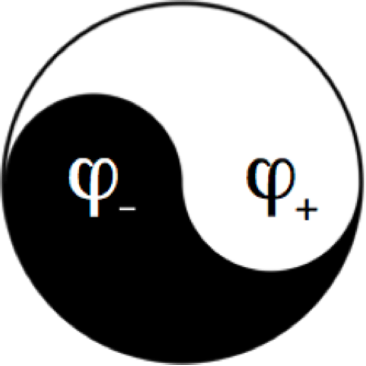At the origin of ALL the continuous equal proportions of the infinite void (0) and the finite point (1) combine geometrically/mathematically to form the quantized virtual dimensional number number field — Pascal’s triangle.
Envision the singularity, the energetic monad, the singular spherical dimensional origin reflecting upon the surrounding void, causing higher dimensions to unfold as they rotate harmonically about the central sphere with the complementary cuboctahedral dipole super-symmetry of the continuous golden proportion — a.k.a. the Fibonacci series.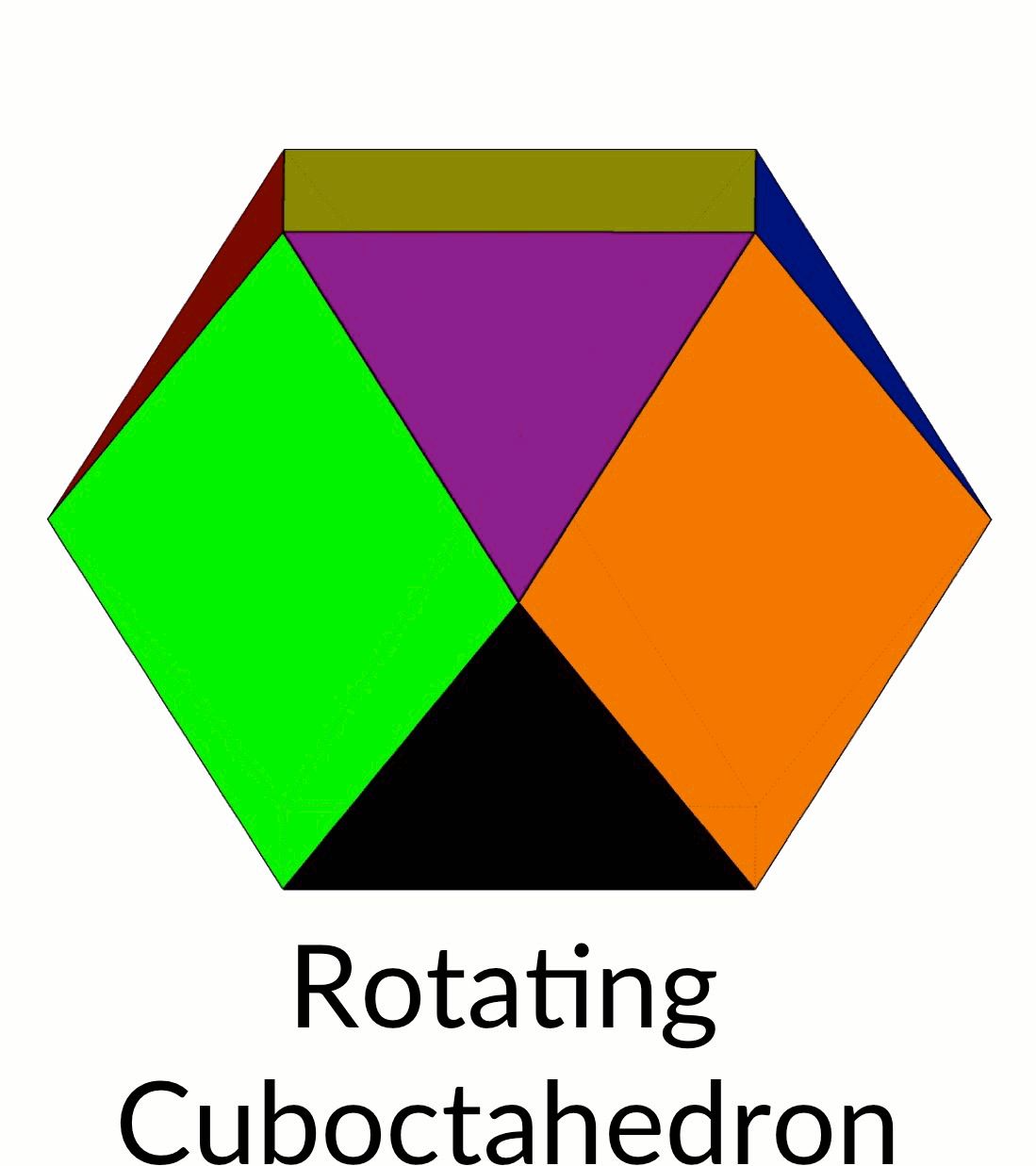Then envision these dimensional super-symmetries of energy (spatial/temporal) and anti-energy (temporal/spatial) constrained to the spatial/temporal perspective and integrated to form the finite atomic particles we observe as the electron and proton — spatial/temporally oriented finite integrals of energy and anti-energy — complementary dipoles.
The wisdom of the ages consolidated and imbued with 21st century hindsight! In an eclectic fusion, Williams merges number theory, geometry, complementary color theory, quantum physics, and ancient philosophy to produce a novel unified field theory. Explore Nature’s complementary dimensional number symmetry.